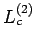Next: 3.2.4 Ansatz Up: 3.2 Formalism Previous: 3.2.2 Multi Scale Method

## 3.2.3 A Conserving Approximation

Both the DMFT and DCA violate certain Ward identities and hence neither of these two methods constitutes a conserving approximation (see ref. (11) for details). This results from the partial momentum conservation violation at each of the internal vertecies as described by their respective Laue functions (Eq. 3.2).

In contrast, the MSMB formalism based on the explicit small cluster vertex (Eq. 3.8 only), restores momentum dependence of the large cluster self-energy constitutes and thus constitutes a conserving approximation for the large cluster. It fulfills both the requirements of 1) the inversion symmetries ofto be preserved and 2) that the two-particle correlation function tends to the single particle Green's functions via the Dyson's equation (for further details see ref. (12)). Furthermore, while the vertex isn't a functional derivative of the large cluster self-energy, this is a sufficient but not a necessary condition for conservation. This MSMB implementation however does not constitute a conserving approximation for the lattice self-energy since we construct it of the large cluster self-energy and not the DCA vertices. Thus, the resulting self-energy breaks the conservation laws with corrections of order(whereis the linear size of the larger cluster).

With the introduction of the-approximation (Eq. 3.9) short ranged correlations are neglected and have to be supplemented as discussed in the subsequent section. In doing so momentum conservation is violated and the resulting MSMB method is once again not a conserving approximation. It should be reiterated however that the necessity of the second (long wavelength) approximation is only temporary until the hurdle of large memory requirements can be met by widely available supercomputers.Next: 3.2.4 Ansatz Up: 3.2 Formalism Previous: 3.2.2 Multi Scale Method
© Cyrill Slezak